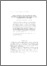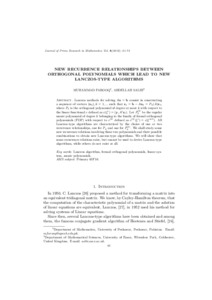# New recurrence relationships between orthogonal polynomials which lead to new Lanczos-type algorithms

Farooq, M and Salhi, A (2012) 'New recurrence relationships between orthogonal polynomials which lead to new Lanczos-type algorithms.' Journal of Prime Research in Mathematics, 8. 61 - 75. ISSN 1817-3462Preview
Text
09.pdf

Download (190kB) | Preview

## Abstract

Lanczos methods for solving Ax = b consist in constructing a sequence of vectors (X ),k = 1,... such that r = b-AX = P (A)r , where P is the orthogonal polynomial of degree at most k with respect to the linear functional c defined as c(ε ) = (y, A r ). Let P be the regular monic polynomial of degree k belonging to the family of formal orthogonal polynomials (FOP) with respect to c defined as c (ε ) = c(ε ). All Lanczos-type algorithms are characterized by the choice of one or two recurrence relationships, one for P and one for P . We shall study some new recurrence relations involving these two polynomials and their possible combinations to obtain new Lanczos-type algorithms. We will show that some recurrence relations exist, but cannot be used to derive Lanczos-type algorithms, while others do not exist at all. k k k k 0 k 0 k k k i i (1) (1) (1) i i+1 (1)

Item Type: Article Q Science > QA Mathematics Faculty of Science and Health > Mathematical Sciences, Department of Jim Jamieson 06 Aug 2013 10:58 07 Apr 2021 10:15 http://repository.essex.ac.uk/id/eprint/7257

### Actions (login required)View Item